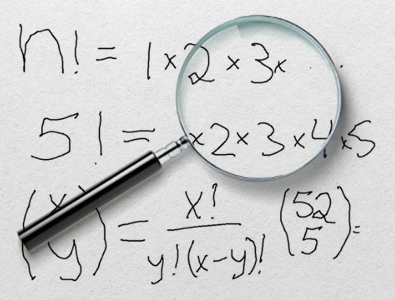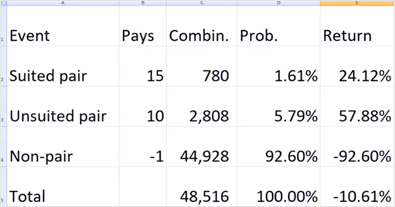## Wizard Recommends

Last Updated: November 9, 2018

# Pair Square Analysis - Blackjack Side Bet

Hi, this is Mike. The purpose of this video will be how to calculate the return to player for the Pair Square side bet in Blackjack. Before I get into 'Bet the Set', otherwise known as a Pair Square, let me quickly go over a couple mathematical functions, which you may already know. n factorial is the number of ways you can arrange n different unique items.

It is simply the product of every integer from one up to n.

For example:

Five factorial would be the number of ways you could arrange five different items, which would be 1×2×3×4×5, which equals 120. Let me also explain the combinations function. That is the number of ways you can choose Y items out of X, in this particular example without regard to order.

For example, the number of ways you might be able to choose three unique side dishes out of eight at El Pollo Loco.

This function...

...equals X factorial divided by Y factorial, divided by X minus Y factorial. I explained why this is true in my probabilities in poker video. An example of this might be, what is the number of ways you can choose five cards out of 52?That is equal to 52 factorial divided by five factorial divided by 47 factorial, which equals 2,598,960. With that housekeeping out of the way, let's move on to part two, where I calculate the combinations of each possible outcome of the Pair Square bet.

Ready to move on to the next step:

which is to calculate the number of combinations of each possible outcome of this bet. The crux of this whole video. Let's assume six decks of cards. For the suited pair, there are 52 different cards in the shoe. 13 ranks times four suits equal 52. They must both be the same.

For example, they must both be the five of diamonds. Once you have chosen your specific card, there are six of those cards in the six-deck shoe.

You must choose two out of those six. Six choose two equals 15, so 52 times 15 is equal to 780. There are 780 ways you can get the big suited pair win. How many ways are there to have an unsuited pair? Well, first, you have to choose your rank of the unsuited pair, for example, fives.

Then you must choose two different suits for that pair, because they must be different. If they were the same, then you would have a suited pair and win more money.

There are four, choose two ways to pick two suits out of four, which equals six. Then, once you have chosen your rank and your two suits, there are six ways to choose each of them out of the six-deck shoe.

For example:

if your two cards were the King of Clubs and King of Spades, there are six of each of them in the six deck shoe. 13 times six times six-squared is equal to 2,808. Finally, the way to lose this bet is to not get a pair.

How many ways can you not get a pair?

Well, you'll have to choose two different ranks out of the 13 available, for example, sevens and tens. Then once you choose your two specific ranks, there are 24 cards of each of those ranks in the whole shoe.

Because each deck has four cards of a specific rank and there are six decks in the whole shoe, so there are 24 aces, for example, on the whole shoe. If our two ranks were, say aces and kings, there are 24 aces and 24 kings.

You pick one out of each of those groups.

13 choose two is, 78 times 24-squared, is equal to 44,928, so there are our combinations. Next, let's move all this over to Excel to see what kind of value we're getting with the Pair Square bet.

Let's go on to the final part of this...

I just showed you the number of combinations of each possible outcome in the Pair Square bet. The most common pay table that you see with six decks is a suited pair of pays 15 to one and an unsuited pair pays 10 to one.Let's calculate the probability of each event - which is really simple. The probability of a suited pair is the number of ways to make a suited pair divided by the total number of ways you can choose two cards out of the 312 and the shoe, which is 40,516. 780 divided by 40,516 comes to about 1.61%.

Using that same formula of the number of combinations of each event divided by the total, we get these other probabilities. A 5.79% of an unsuited pair, and in 92.6% of getting two different ranks.

Next...

...the way I like to analyze a bet is to simply multiply the win times the probability. The return from a suited pair is 15 the win times the probability of getting one, 1.61% which comes to-- Let's make that bigger for you, 24.12%. Using that same formula, we see that the return from an unsuited pair is 57.88%, and the return from a non-pair is a negative 92.6%.

What this means is that if the player-- that's a dollar.

For example: he can expect to get back 24.12 cents from his suited pairs, 57.8 cents from his unsuited pairs, but lose 92.6 cents from his non-pairs. To get the overall value to the player, we just take the sum of that and we get negative 10.61%.

That means...

that the player can expect to lose 10.61% of all the money he bets on the Pair Square. Another way of putting that is that the house advantage is 10.61%. In other words, for every dollar bet on this, the player can expect to get back about 89.39 cents, and 10.61 cents goes to the casino.

To put this in perspective: the house advantage in blackjack itself can be as low as 0.28%. Even with bad rules, it's more like 0.6%. Here you are with this house advantage much under 1%, assuming a blackjack pays three to two. Which you should strive to get every chance you get, and then this house advantage of almost 11%.

It just goes to show what I have said thousands of times that side bets are sucker bets. This is a perfect example of that. I recommend that you avoid this side bet as well as every side bet if you can.

Thanks for watching guys, and I will see you in my next video.